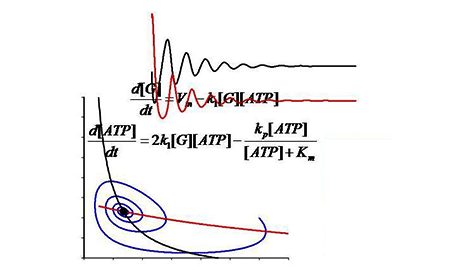ECEP VIRTUAL CAMPUS

# Dynamical Modeling Methods for Systems Biology

An introduction to dynamical modeling techniques used in contemporary Systems Biology research.## Categories

We take a case-based approach to teach contemporary mathematical modeling techniques. The course is appropriate for advanced undergraduates and beginning graduate students. Lectures provide biological background and describe the development of both classical mathematical models and more recent representations of biological processes. The course will be useful for students who plan to use experimental techniques as their approach in the laboratory and employ computational modeling as a tool to draw deeper understanding of experiments. The course should also be valuable as an introductory overview for students planning to conduct original research in modeling biological systems.

This course focuses on dynamical modeling techniques used in Systems Biology research.  These techniques are based on biological mechanisms, and simulations with these models generate predictions that can subsequently be tested experimentally. These testable predictions frequently provide novel insight into biological processes. The approaches taught here can be grouped into the following categories: 1) ordinary differential equation-based models, 2) partial differential equation-based models, and 3) stochastic models.

## Course Syllabus

Topics covered include:
• Modeling in Systems Biology
• Computing with MATLAB
• Dynamical systems tools to analyze behavior of mathematical models
• Modeling emergent Properties: bistability in biochemical signaling
• Modeling the cell cycle
• Modeling electrical signaling in neurons
• Mathematical models of electrical signals that propagate in space and time
• Modeling the spatial dependence of intracellular signaling
• Mathematical models of processes in which randomness is important

## Recommended Background

Two semesters of undergraduate-level calculus are required.

Review articles and selected original research articles are discussed in the lectures and can enhance understanding, but these are not required to complete the course. All materials will be from open access journals or will be provided as links to e-reprints, so there will be no cost to the student.

## Course Format

Each class session will consist of an approximately one hour lecture divided into multiple shorter segments, which will include solving a sample problem to illustrate the method being taught in the lecture.

For evaluation, students will be given assignments that will require: 1) implementing a mathematical model; 2) performing simulations and/or analysis of the model; and 3) interpreting the biological implications of the results in multiple choice format. The average of the six assignments will be used to calculate overall course grade.

## FAQ

• Will I get a Statement of Accomplishment after completing this class?

Yes. Students who successfully complete the course will get a Statement of Accomplishment signed by the Course Director.

• Starts August 18, 2015

### Course at a Glance

10 weeks of study 2-4 hours/week English English & Vietnamese subtitles